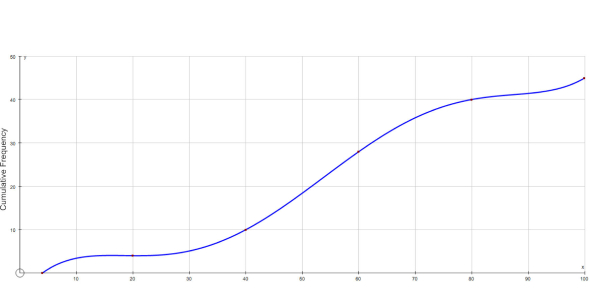Cs 10 Cumulative Test

14 Questions | Total Attempts: 48SettingsUCR CS 10 Cumulative Review

• 1.
#include [iostream] using namespace std; // What goes here? {     return 0; }
• 2.
What is the most appropriate data type with which to store the value 90032 Beers on the Wall?
• 3.
Which of the following is a declaration statement.
• A.

Foo = "bar";

• B.

Cin >> foo;

• C.

String foo;

• D.

Foo += "bar";

• 4.
Which of the following is an assignment statement?
• A.

Foo = "bar";

• B.

String foo = "bar";

• C.

String foo;

• D.

If (foo == "bar") { cout

• 5.
Which of the following has a syntax error? 1. string H, K; cout << "Enter your hat's name and your kat's name: "; cin >> h >> k; 2. double s, q; cout << "Enter your first and last name: "; cin >> s >> q; 3. string s, q; cout << "Enter your first and last name: "; cin >> s >> q; 4. int i; cout << "Enter the number of shoes you have: "; if (i == 0)     cout << "I'm very sorry."; else if (i > 1)     cout << "Can I borrow one?"; cout << endl;
• A.

1

• B.

2

• C.

3

• D.

4

• 6.
Int a = 5; if (false) {     if (a == 5)     {         cout << "foo ";     } } cout << "bar"; What is the output of the above program?
• 7.
String player = "Michael"; cout << player << "," << setfill('-') << right << setw(10) << player << ","; player = "Kobe"; cout << setw(10) << player << setfill('*'); What is the output of the above program. Make sure to be exact!
• 8.
#include [iostream]        // 1 using namespace std;       // 2 int main()                 // 3 {                          // 4     string s, p;           // 5     cin >> s >> p;         // 6     if (s = "foo")         // 7     {                      // 8         cout << p; cout << // 9         "Foo";             // 10     }                      // 11     else                   // 12     {                      // 13         cout << s;         // 14     }                      // 15 }                          // 16 Which of the above line(s) contain an error? If multiple lines have errors, seperate each line with a comma (but no space). So if 14 and 15 had errors, you would write 14,15.
• 9.
String s = "Hello"; s += "World!"; int p = s.find('o'); cout << s.substr(0, p); What is the output of the above code fragment?
• 10.
Int i = 5; while (i > 0) {     i -= 2;     cout << i;     i++; } What is the output of the above code fragment?
• 11.
For (int i = 0; i < 3; i++)     for (int j = 0; j < 3; j++)         cout << i * j; What is the output of the above code fragment?
• 12.
True && !(true || false) && (false || (true && true))
• A.

True

• B.

False

• 13.
Int foo() {     return 4; } int bar(double z) {     return static_cast[int](z / foo()); } int qux(int a, int b) {     return (bar(a) * foo()) % b; } int main() {     cout << qux(4, 9);     return 0; } What is the output of the above program?
• 14.
Int foo(int & a, int & b) {     if (a > b)         b = a;     else         a = b;     return a + b; } int main() {     int a = 5, b = 4;     cout << foo(a, b) << a << b;     return 0; } What is the output of the above program?
Related TopicsBack to top